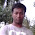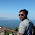# How to convert string to integer in C

In this post, I am going to show you how to convert a string to a single integer number. Sometimes we need to take input the number as a string and then need to convert them into a single integer. I will show you two ways to convert string to integer. First we will convert manually and then we will use library function to convert string to integer.

## Manually convert string to integer:

In the string numbers are stored in character format. So if we deduct character '0' from each element we will get the decimal value. For Here is the table of ASCII characters (number) and their decimal value:

 Character Decimal Value 0 48 1 49 2 50 3 51 4 52 5 53 6 54 7 55 8 56 9 57
So, Character ‘6’ – ‘0’ = 54 – 48 = 6 ( decimal 6 ). This theory is applied to the following example:

`#include <stdio.h>#include <string.h>int main(){	char num;	int dec = 0, i, j, len;	printf("Enter a number: ");	gets(num);	len = strlen(num);	for(i=0; i<len; i++){		dec = dec * 10 + ( num[i] - '0' );	}	printf("%d", dec);	return 0;}`

## Convert Using built in library function:

We have a built in function in C header file stdlib.h to convert string to integer. And the function is int atoi(const char *str) . This function returns converted intger number on success.

Here is an example:

`/* atoi example */#include <stdio.h>#include <stdlib.h>int main (){  int i;  char num;  printf ("Enter a number: ");  gets(num);  i = atoi (num);  printf ("The value entered is %d.\n",i);  return 0;}`

Output of the program:

Share:

#### 6 comments :

1.tell me how to to convert a int to string without suing any predefined function

2.thanks

3.4.Thank you...

5.The output does not work for string lengths more than 10...

1.it does not fit into int value

Spam comments will be deleted. :)